## Excel IF Function## What is the IF function?

One of the most used logical functions in Excel, the IF Function, is used to check whether a condition is met, and returns one value if true and another value if false.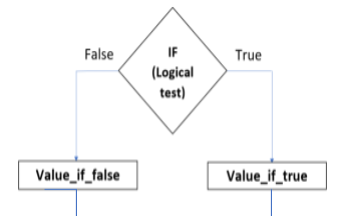The IF syntax in Excel is

=IF(logical_test,value_if_true, value_if_false)

The above syntax has 3 arguments – logical_test; value_if_true; value_if_false; but only the first one is obligatory, the other two are optional.

Check Out | Excel Club## The Simple IF function

To understand the simple IF function, let us take an example.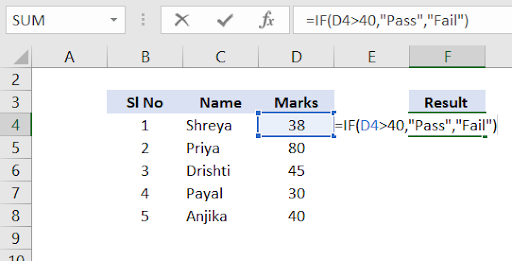Logical_test: In the example, a candidate has “passed” only if they score more than 40 marks.

Value_if_true: If the value is true – that is, if the candidate has been awarded more than 40 marks, the result is “pass”.

Value_if_false: If the value is false – that is, if the candidate has been awarded less than 40 marks, the result is “fail”.

Always Remember:

• In the logical argument: you can specify a text value, date, number or any comparison operator.
• For example, your logical test can be D4= “Fail”, D4=40, D4>40 and so on
• Use quotation marks if you want the result to be text instead of a number.
• If the value_if_true argument is omitted in your formula, the IF function returns zero (0) when the condition is met.
• If you do not want to add any value for the false logic, simply add 2 quotation marks - “”
• The easiest way to make a proper formula is to write down the condition first, then incorporate it in the logical_test argument of the IF function

Example:

Condition: D8>40

IF formula: IF(D8>40, “Pass”, “Fail”)

## The IF with AND function

When a situation arises where you need to do something specific if two or more conditions are TRUE, you can use the IF function in combination with the AND function to evaluate the conditions. If the condition evaluates to FALSE, the result is changed.

The IF with AND function syntax is IF (AND (condition1, condition 2, …), value_if_true, value_if_false)

Let us take an example: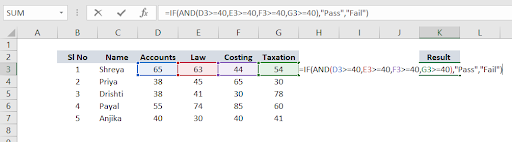Logical_test: in the above example is that the student must have been awarded greater than or equal to 40 marks in all the 4 papers. Only if this condition is met, the student is considered to have passed.

Value_if_true: If the value is true – that is, the candidate has been awarded more than or equal to 40 marks in ALL the subjects, then the result is “pass”.

Value_if_false: If the value is false – that is, if there is 1 subject in which the candidate has not secured 40 marks (less than 40 marks), she is considered as “fail”.

## The IF with OR function

The OR function is a logical function to test multiple conditions at the same time. It is used to compare two values or statements. The OR function can be used to extend the functionality of the IF function. It can be used as the logical_test for an IF function.

The IF with OR function syntax is

IF(OR(logical 1, logical 2, …), value_if_true, value_if_false)

Let us take an example: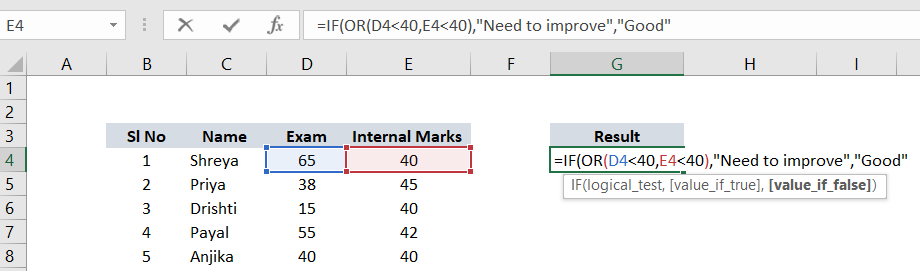Logical_test: The student must have received less than 40 marks in either the internals or the exam.

Value_if_true: The value if true – that is if the student has scored less than 40 in either the exam or the internal, then the result should be “Need to improve”.

Value_if_false: The value if false – that is if the student has scored greater than or equal to 40 marks in both the actual exam and the internal, then the result should be “Good”.

Always Remember:

• Don’t forget to add a bracket after entering the AND/OR condition
• Don’t forget to add a comma after the entering the AND/OR condition and the bracket.
• The condition you will be adding after the AND/OR condition is for the IF condition. You merely need to add the “value_if_true” and “value_if_false”
• You are not limited to using only two AND/OR functions in your Excel formulas. Your formula may include as many as 255 arguments, and the total length of the formula should not exceed 8,192 characters.

### Difference between ‘IF with AND’ and ‘IF with OR’

When the IF AND function is being used all the conditions must be met for Excel to return the value as true. But in case of the IF OR function, Excel returns the value as true even if only one condition is met.

## Nested IF

The Nested IF function is used when the user needs to test for more than one condition, then take one of the several actions, depending on the result of the results.

This function includes embedding or “nesting” one IF function inside another.

It allows you to test multiple criteria and increases the number of possible outcomes.

The Nested IF syntax is

IF(logical_test, value_if_true, IF(logical_test, value_if_true, IF(logical_test, value_if_true, value_if_false)))

Let us take an example :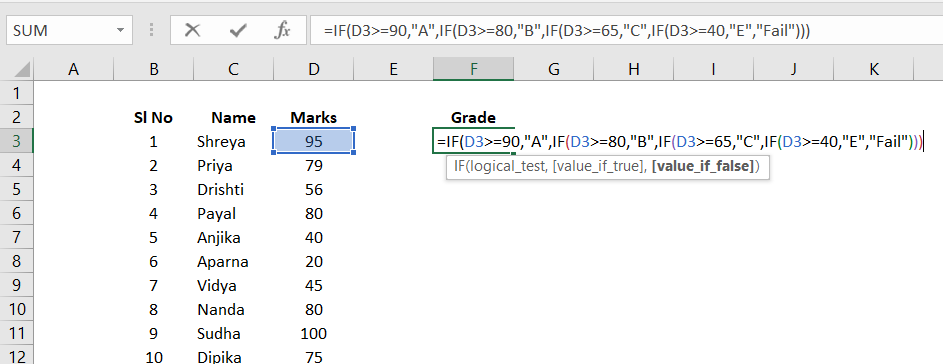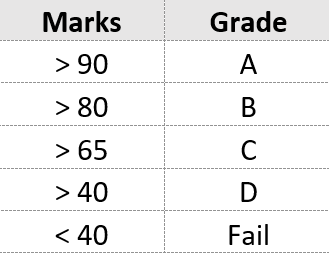Logical_test: The grading system given alongside was used as the logical test in the above example.

Value_if_true: Based on the above logical test, if the candidate falls into any one of the marking schemes, the grade will be awarded accordingly

Value_if_false: If the candidate does not fall into any of the marking scheme that is, she has scored below 40 marks the result will be declared as fail.

## Cell Referencing in Logical Test

Generally, we type the logical test manually. Another way to add an argument to your logical test is via cell referencing. This makes it more flexible and easier – that is if you want to change the value of the argument in the logical test, you can just change the value in the cell you have given reference to and the formula gets automatically adjusted.

Remember, to always FREEZE/FIX the cell reference by adding the dollar sign (\$) before the column and row number. You can also press the F4 key to freeze/fix the cell reference

Let us take an example to understand this,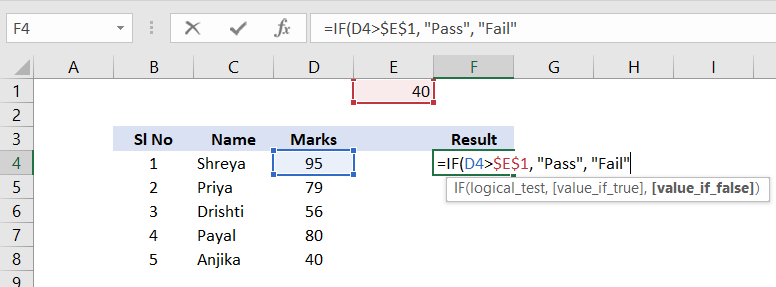In the above example, Cell E1 has been fixed with the numerical value 40

## Logical Operators to use with ‘IF’

A logical operator is used in Excel to compare two values. The following table explains what each of them does and illustrates the theory with formula examples.

 Operator Meaning Example Description = Equal to =IF (B2=5, “ok”, “change”) If the number in the cell is 5, the formula returns “ok”; otherwise it displays “change” > Greater than =IF(B2>5, “ok”, “”) If the number in the cell is greater than 5, the formula returns “ok”; otherwise an empty cell is displayed < Lesser than =IF (B2<5, “ok”,) If the number in the cell is lesser than 5, the formula returns “ok”; otherwise it returns 0 >= Greater than equal to =IF(B2>=5,, “ok”) If the number in the cell is greater than equal to 5, the formula returns 0; otherwise it displays “ok” <= Lesser than equal to =IF(B2<=5, “Correct”, “Wrong”) If the number in the cell is lesser than or equal to 5, the formula returns “Correct”; otherwise it displays “Wrong” <> Not equal to =IF(B2 <> 5, “Good”, “Bad”) If the number in the cell is not equal to 5, the formula returns “Good”; otherwise it displays “Bad”

Shreya Nayak